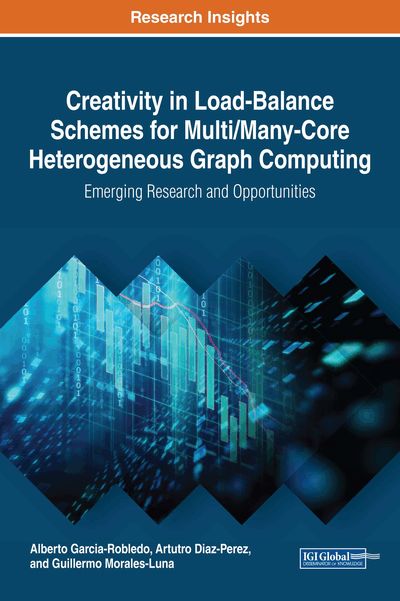# Core Kernels for Complex Network Analysis

DOI: 10.4018/978-1-5225-3799-1.ch002

## Abstract

The emergence of Network Science has motivated a renewed interest in classical graph problems for the analysis of the topology of complex networks. For example, important centrality metrics, such as the betweenness, the stress, the eccentricity, and the closeness centralities, are all based on BFS. On the other hand, the k-core decomposition of graphs defines a hierarchy of internal cores and decomposes large networks layer by layer. The k-core decomposition has been successfully applied in a variety of domains, including large graph visualization and fingerprinting, analysis of large software systems, and fraud detection. In this chapter, the authors review known efficient algorithms for traversing and decomposing large complex networks and provide insights on how the decomposition of graphs in k-cores can be useful for developing novel topology-aware algorithms.
Chapter Preview
Top

## Characterizing The Topology Of Complex Networks

Network Science was initiated in the late 1950’s and early 1960’s with the works of Erdös and Rényi (1959) on random graphs. However, during late 1990’s interest in complex networks exploded with the seminal works of Watts and Strogatz on small-world networks (Watts and Strogatz, 1998) and Barabási and Albert on scale-free graphs (Barabási and Albert, 1999). They showed that complex networks encode intriguing properties in their topology that reveal important details of their functional organization and the underlying real-world phenomena.

Many complex network metrics have been proposed in literature (Newman, 2003; Costa et al., 2007) to characterize the topology of complex networks.

Complex network metrics can be roughly grouped into two classes: structural and scaling metrics. On the one hand, structural metrics summarize information about the global structure of the graph, or the local organization or importance of vertices. On the other hand, the scaling of some structural metrics with the degree of vertices can reveal non-trivial high-level properties of graphs, such the scale-free form of the graph degree distribution.

Complex network metrics give information about individual elements of a graph, for example, the importance of a vertex in terms of its degree, vertex distance to other nodes, or the properties of a vertex neighborhood. Complex network metrics can also give information about the whole graph; for example, the longest shortest path distance between any two vertices (diameter), the maximum number of neighbors of any vertex, or how much the degree distribution follows a scale-free form. Finally, the scaling with the degree of some metrics, such as the clustering or the rich-club coefficient, reveals, for example, if a degree distribution follows a scale-free form or the assortativity of a graph.

## Complete Chapter List

Search this Book:
Reset